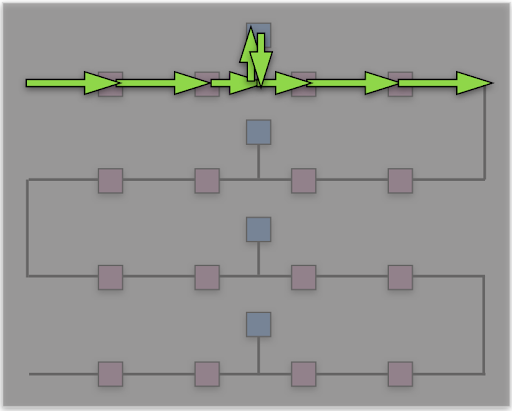# Lesson 4: For Loops with GarbageRider

### Introducing GarbageRider

Hi, my name is GarbageRider. I am a robot that collects garbage in the streets. I’ll need instructions to find all the garbage cans. Then we’ll also try to find all of the dumpsters and clean those up as well. We’ll need an efficient path so we can get this job done as quickly as possible!

### Introduction

In this lesson, you will learn about for loops. A for loop runs a set number of times. Earlier you learned about while loops. In the example below the while loop moves forward and turns right forever. The for loop will move forward and turn right, repeated three times.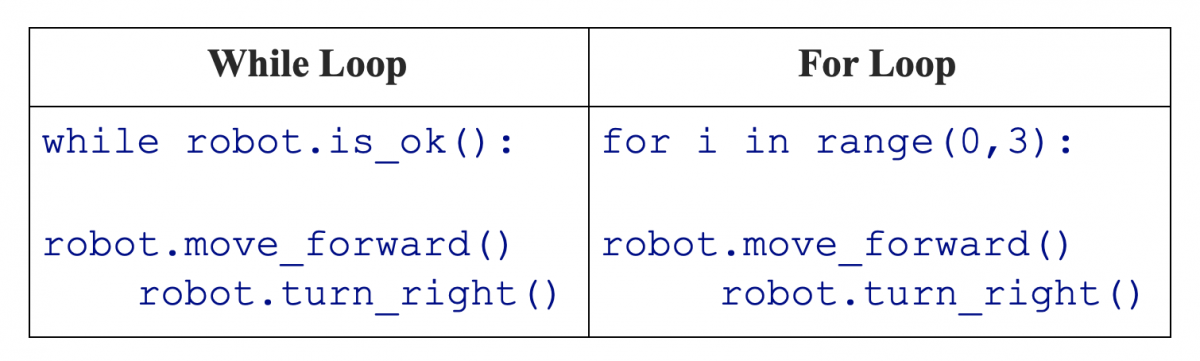In the for loop above there is an index i. The for loop runs three times so the index i will take on the values 0, 1, and 2. Notice that since we start with index i = 0, we never get to index i = 3.

### Getting Started

1. Start the simulation.
2. The diagram below shows the locations of the trash bins. We will try to collect all the spots marked as pink first.
3. GarbageRider cannot be moving when it picks up a trash bin. GarbageRider must stop at each pickup point.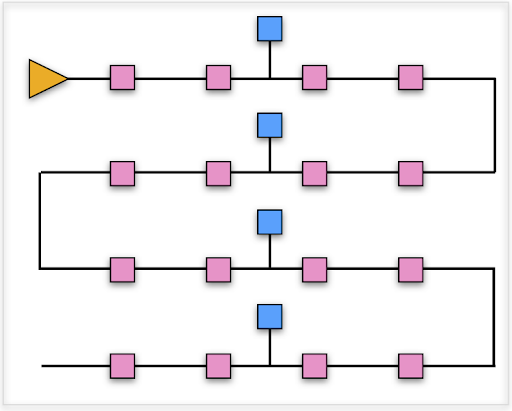### Robot Commands

1. robot.move_distance(X) – Moves the robot forward X meters and then stops.
2. robot.rotate_angle(X) – Rotates the robot around its center X radians. Negative X means rotate counter-clockwise (CW). Positive X means rotate clockwise (CW).

### Checkpoints

Checkpoint #1 – First Collection

• Let’s try the simplest thing and move forward one meter to the first pickup.
• robot.move_distance(1.0) Run the simulation and examine the robot’s motion. We can represent this algorithm as shown below.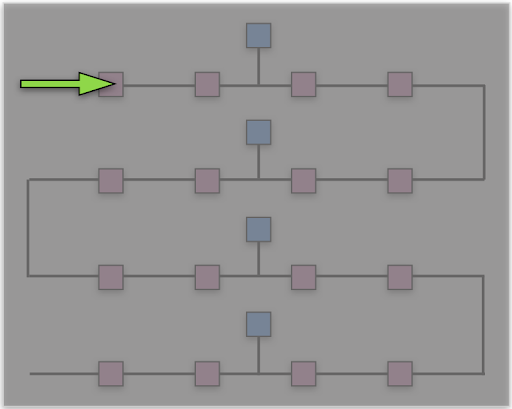Checkpoint #2 – Completing the First Street

• Now copy your move command so it appears five times, as shown below

robot.move_distance(1.0)

robot.move_distance(1.0)

robot.move_distance(1.0)

robot.move_distance(1.0)

robot.move_distance(1.0)

Run the simulation and examine the robot’s motion. We can represent this algorithm as shown below.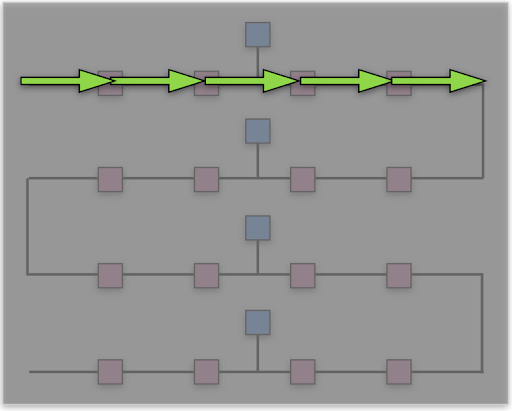• When programming, it’s not a good design to have things repeat over and over. Can you think of reasons why duplicating code is not a good design? See if you can rewrite your code to use a for loop. The Introduction above has an example of a for loop you can use as a starting point.

Checkpoint #3 – Completing the First Turn

• You can make a left turn using the code below.
robot.rotate_angle(math.pi/2.0)
What would a right turn look like?
• Using the diagram below as a guide, try adding rotate and move commands to complete the first turn.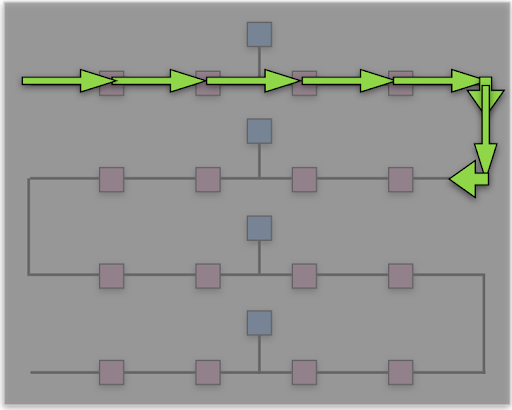• Run the simulation and examine the robot’s motion.

Checkpoint #4 – Completing the Second Turn

• Using the diagram below as a guide, try adding code to complete the second turn. For your first attempt, you can simply copy all of the code you have so far so it repeats. But you’ll need to change the sign of some rotate_angle calls.• Run the simulation and examine the robot’s motion.

Could we write a shorter code instead? Instead of repeating all of the code twice, what if we added a second for loop with a range of 2? Here is an example to help you get started.

for j in range(0,2):

for i in range(0,5):

# more of your code here

•    Notice that the first loop uses index j and the second loop uses index i. Now you have a for loop inside of another for loop. Make sure everything has the proper indentation. The change in sign for the rotate_angle calls presents a problem. Can you use the index j to fix it with conditionals? You’ll need to change the sign of the angle depending on whether j is 0 or 1.

Checkpoint #5 – Collecting all the Pink Trash

• Using the diagram below as a guide, try adding code to collect all of the pink locations. For a first try you can try repeating all of your previous code twice. Or just go for it and change your for loop with index j from range 2 to range 4. Can you make the rotate_angle calls work with the proper signs?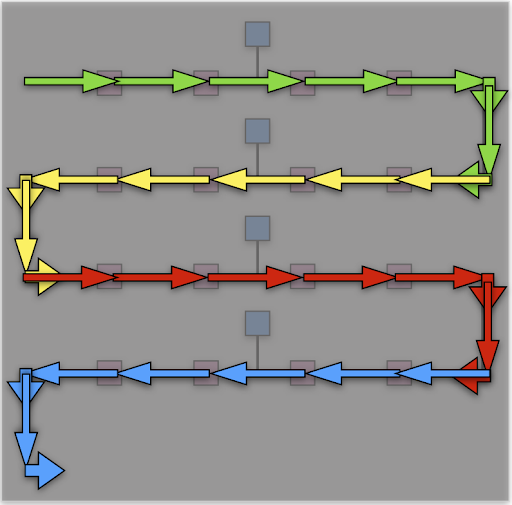• Run the simulation and examine the robot’s motion.
• How compact can you make your code? Can you make it work with less than 10 lines?

### Challenge

Now try to modify your code so it collects all the pink and blue pickup locations. To get started, consider the path in the image below. Try to think of ways to make your code as compact as possible.